Question Pool Real Numbers

# Real Numbers - SAMAGRA Question Pool & Answers | Class 9 English Medium

Kerala Syllabus SAMAGRA SCERT SAMAGRA Question Pool for Class 9 English Medium Maths Real NumbersQn 1.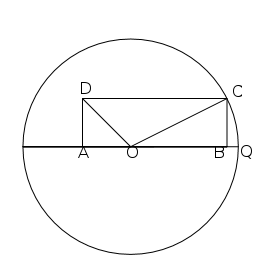In the figure ABCD is a rectangle. AB=3, OA= AD=1, O represents the number Zero . Then

a) Write the numbers represents A and B

b) The circle of radius OC and centre O meet the line at Q. Which is the number represents Q ?

Get Free Study Materials + 1 Week Free Trial of BrainsPrep Class 9 English Medium Tuition

Qn 2.

Mark the number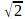and -on the number line.

Get Free Study Materials + 1 Week Free Trial of BrainsPrep Class 9 English Medium Tuition

Qn 3.

Point A on a number line represents the number 5.

a) Which number is represented by the point 4 units right to A.

b) Which number is represented by the point 7 units left to a.

a)

Get Free Study Materials + 1 Week Free Trial of BrainsPrep Class 9 English Medium Tuition

Qn 4.

-2, 10 are represented by the points A and B on the number line. Then

a) What is the length of AB?

b) C and D are two points on AB such that AC: CB=1 : 2 and AD:DB=2:1

Then which are the numbers representing the points 'C' and 'D'

Get Free Study Materials + 1 Week Free Trial of BrainsPrep Class 9 English Medium Tuition

Qn 5.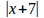=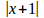find x ?

Get Free Study Materials + 1 Week Free Trial of BrainsPrep Class 9 English Medium Tuition

Qn 6.

If x=5, y=-2 then

a) Find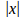,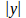,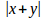,+and check whether=+b) Write two number x and y such that=+Get Free Study Materials + 1 Week Free Trial of BrainsPrep Class 9 English Medium Tuition

Qn 7.

If =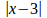=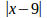, find x?

x is at equal distance from 3 and 9

The number representing x is the midpoint of the distance between 3 and 9

x =

Get Free Study Materials + 1 Week Free Trial of BrainsPrep Class 9 English Medium Tuition

Qn 8.

Find the numbers on the number line which are 5 uniis away from the point representing 2.

7

Get Free Study Materials + 1 Week Free Trial of BrainsPrep Class 9 English Medium Tuition

Qn 9.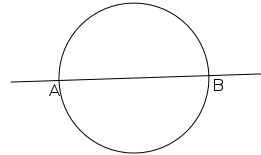A circle is a drawn with centre on a number line. AB is its diameter. The points A and B represent-2 and 7.

b) Find the number representing the centre of the circle?

Get Free Study Materials + 1 Week Free Trial of BrainsPrep Class 9 English Medium Tuition

Qn 10.

a) What is the distance between the points representing the numbers -2 and 10 on the number line?

b) Which number is represented by the point that divides this distance in the ratio 1:2 ?

Get Free Study Materials + 1 Week Free Trial of BrainsPrep Class 9 English Medium Tuition

Qn 11.

The point k on the number line represents -5. L is a point on the right of K at a distance of 9 units.

a) Which number represents the point L?

b) The points X and Y divide the length KL into 3 equal parts . Which are the numbers representing these points?

a) 4

b) X represens   -2

Y represents 1

Get Free Study Materials + 1 Week Free Trial of BrainsPrep Class 9 English Medium Tuition

Qn 12.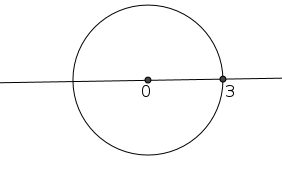A Circle drawn with Zero on the number line as its centre passes through the point representing 3.

b) Which number represents the other point?

b) -3

Get Free Study Materials + 1 Week Free Trial of BrainsPrep Class 9 English Medium Tuition

Qn 13.

Point A represents the number 5 on a number line

a) Which number is represented by the point 4 units right to A.

b) Which number is represented by the point 7 units left to A

a) 9

b)

Get Free Study Materials + 1 Week Free Trial of BrainsPrep Class 9 English Medium Tuition

Qn 14.

The area of a rectangle is 6 sq. Unit.

a) Write two natural numbers that can be the length of its sides.

b) Write two irrational numbers that can be the length of its sides.

Get Free Study Materials + 1 Week Free Trial of BrainsPrep Class 9 English Medium Tuition

Qn 15.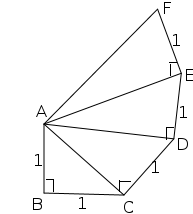ABC is a right angled triangle with AB=BC=1 unit. ACD is a right angled triangle formed by drawing a perpendicular of length 1 unit to the hypotenuse. ADE and AEF are right angled triangles formed by the same process. Which among them have hypotenuse with irrational length.

ABC

Get Free Study Materials + 1 Week Free Trial of BrainsPrep Class 9 English Medium Tuition

Qn 16.

Four squares and their areas are given. Which among them have length of sides irrational?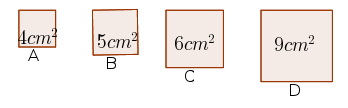B

C